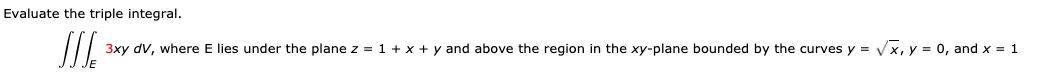# Evaluate the triple integral. 3xy dV, where E lies under the plane z = 1 + x + y and above the region in the xy-plane bounded by the curves y = Vx, y = 0, and x = 1

Questionhelp_outlineImage TranscriptioncloseEvaluate the triple integral. 3xy dV, where E lies under the plane z = 1 + x + y and above the region in the xy-plane bounded by the curves y = Vx, y = 0, and x = 1 fullscreen

### Want to see this answer and more?

Experts are waiting 24/7 to provide step-by-step solutions in as fast as 30 minutes!*

*Response times may vary by subject and question complexity. Median response time is 34 minutes for paid subscribers and may be longer for promotional offers.
Tagged in
Math
Calculus

### Integration